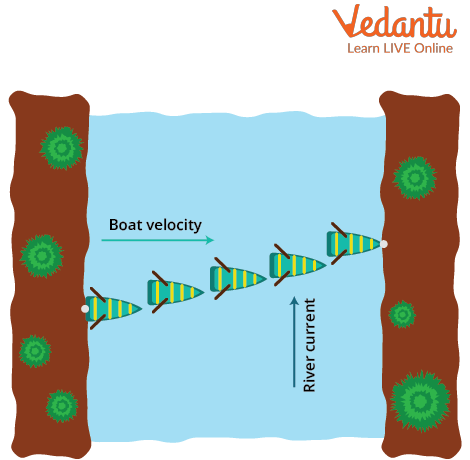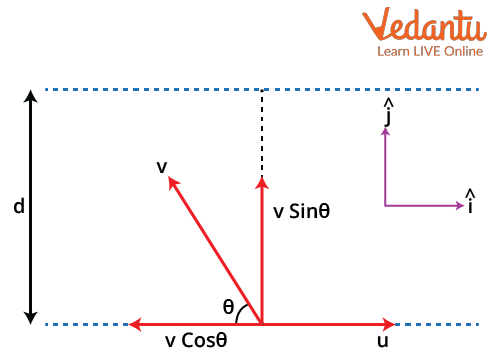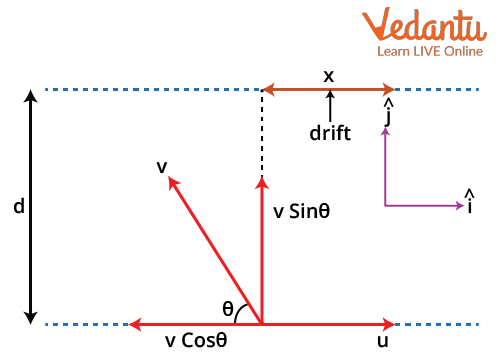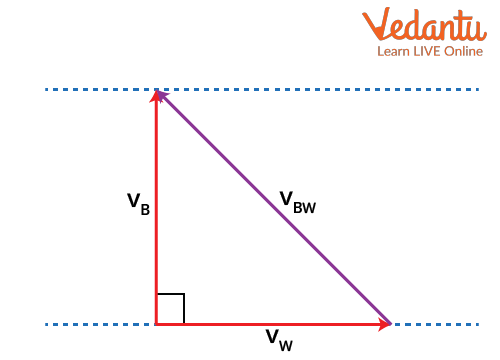Courses
Courses for Kids
Free study material
Free LIVE classes
More

# River Boat Problem in 2D From Relative Velocity - JEE Important TopicLIVE
Join Vedantu’s FREE Mastercalss

## A Brief Introduction to Relative Velocity

We come into situations when one or more objects move in a non-stationary frame with respect to another observer. For example: a boat crossing a fast-flowing river or an aeroplane flying in the air encountering wind. In all these cases, we must consider the medium's effect on the item to characterise the object's whole motion. We calculate the relative velocity of the object while doing so, taking into account the particle's velocity as well as the velocity of the medium. Since velocity is a vector, the calculations of relative velocity include vector algebra. We’ll look at the problem of relative velocity in more detail throughout this article and discuss river velocity, river boat problems and solutions to relative velocity.

## Examples and Mathematical Formulation of Relative Velocity

We all have encountered relative velocity at some point. Some classic examples of relative velocity include situations where, while travelling by automobile, bus or train, you may see that the trees, buildings and other objects outside are moving backwards. Is it true, however, that they are going backwards? No! You're completely aware that your vehicle is moving while the trees remain motionless on the ground. But, if that's the case, why are the trees travelling backwards? Also, even though they are moving, your fellow passengers appear to be motionless to you.

This is where the concept of relative velocity comes into play. The passengers appear motionless to you because they are at rest, relative to you. However, to someone on the ground who isn’t moving, your fellow passengers are in motion, relative to them. You are also in motion relative to someone on the ground. Although the person on the ground might not be moving, according to you they are moving backwards and they have a velocity relative to you.

Let’s consider two objects and name them objects A and B. Suppose object A has velocity vA and object B has velocity vB and they are moving relative to some common stationary frame of reference. This frame of reference could be anything; the ground, a lamppost, a bridge, etc.

Relative velocity is just the difference between the velocities of the objects. Already emphasised before, this difference is not the ordinary difference because velocities are vectors. So, they will follow the rules of vector algebra.

The velocity of object A relative to B is represented as vAB. The formula for this is:

$v_{A B}=v_{A}-v_{B}$

Similarly, the velocity of object B relative to A is represented by vBA  and its formula is:

$v_{B A}=v_{B}-v_{A}$

From the expressions of vAB and vBA, we can say that they both are additive inverses of each other. This means that:

$v_{A B}=-v_{B A}$

This means that vAB has a direction that is opposite to vBA. Even though they have opposite directions, their magnitude remains the same.

$\left|v_{A B}\right|=\left|v_{B A}\right|$

## River Boat Problem in 2D

When a boat is moving through a river, it is affected by the velocity of the water. The directions of the velocities of the boat and the river are usually different. The motion of the boat is influenced by the relative velocity between them. As usual, the concept of relative velocity will be applied and then the problems will be solved accordingly.

If a motorboat was heading straight across a river, it would not reach the point exactly opposite to where it started from. This is due to the river current that influences its motion.

Let’s suppose that we have a motorboat which is moving with a velocity of 6 $\dfrac{m}{s}$ directly across the river. If the river has a velocity downstream, the actual resultant velocity of the motorboat will not be the same as it was initially. The velocity of the boat will be a bit more than 6 $\dfrac{m}{s}$ and it will not be in the direction straight across the river, but at some direction downstream with a certain angle.

The shortest path in the river boat problems is when the boat moves perpendicular to the river current. This whole situation will become clear with some numerical examples that we’ll see in the next section.

To solve any river boat problem, two things are to be kept in mind.

1. A boat's speed with respect to the water is the same as its speed in still water.

2. The velocity of the boat relative to water is equal to the difference in the velocities of the boat relative to the ground and the velocity of the water with respect to the ground. If vBW is the velocity of the boat with respect to the water, and vB, vW are the velocities of the boat and water with respect to the ground respectively, then:

$v_{B W}=v_{B}-v_{W}$Boat and River Current Velocities

## Crossing The River in The Shortest TimeSchematic Diagram of a Boat Going Across a River

Suppose that u is the velocity of the river and v is the velocity of the boat. The boat moves at some angle $\theta$ with respect to the horizontal as shown in the figure. The total velocity of the boat will be the sum of the velocity of the boat with respect to the ground and the velocity of the river. This will be given as

\begin{align} &v_{b}=\vec{v}+\vec{u} \\ \\ &v_{b}=-v \cos \theta \hat{i}+v \sin \theta \hat{j}+u \hat{i} \\ \\ &v_{b}=(-v \cos \theta+u) \hat{i}+v \sin \theta \hat{j} \end{align}

The boat needs to move in the vertical direction in order to make it across the river so only the vertical component of the velocity will be used in getting it across the river. The vertical component is $v\sin{\theta}$. The width of the river is d and so the time taken to cross the river will be

$t=\dfrac{d}{v\sin{\theta}}$

For a minimum time

$\sin{\theta}=1$

$\theta=90^{\circ}$

This means that the minimum time to cross the river will be

$t_{min}=\dfrac{d}{v}$

## Crossing the River Along the Shortest PathSchematic Diagram of A Boat Going Across The River With Some Drift

For the boat to go across the river along the shortest path, the drift x should be minimum or more precisely zero. The drift x will be zero when the velocity in the i direction will be zero. This means that

\begin{align} &u-v \cos \theta=0 \\ \\ &v \cos \theta=u \\ \\ &\cos \theta=\dfrac{u}{v} \\ \\ &\theta=\cos ^{-1}\left(\dfrac{u}{v}\right) \end{align}

So in order for the boat to go along the shortest path it has to go at an angle of $\theta=\cos^{-1}\left(\dfrac{u}{v}\right)$ with the vertical.

The time for the shortest path will be given as

$t=\dfrac{d}{v\sin{\theta}}$

Now we have $\cos{\theta}=\dfrac{u}{v}$ and we know that

\begin{align} &\sin ^{2} \theta+\cos ^{2} \theta=1 \\ \\ &\sin ^{2} \theta=1-\cos ^{2} \theta \\ \\ &\sin \theta=\sqrt{1-\cos ^{2} \theta} \end{align}

Putting the value of $\sin{\theta}$ will give

\begin{align} &\sin \theta=\sqrt{1-\left(\dfrac{u}{v}\right)^{2}} \\ \\ &\sin \theta=\sqrt{1-\dfrac{u^{2}}{v^{2}}} \\ \\ &\sin \theta=\sqrt{\dfrac{v^{2}-u^{2}}{v^{2}}} \\ \\ &\sin \theta=\dfrac{\sqrt{v^{2}-u^{2}}}{v} \end{align}

Inserting this in the expression for time gives

\begin{align} &t=\dfrac{d}{v\left(\dfrac{\sqrt{v^{2}-u^{2}}}{v}\right)} \\ &t=\dfrac{ d}{\sqrt{v^{2}-u^{2}}} \end{align}

This is the time taken along the shortest path.

## Numerical Examples of Relative Velocity River Boat Problems

Example 1: A boat has a velocity of 10$\dfrac{km}{hr}$ in still water and it crosses a river of width 2 km. If the boat crosses the river along the shortest path possible in 30 minutes, calculate the velocity of the river water.

Solution:

Since it is given that the boat crosses the river in the shortest path possible, it means that the boat moves perpendicular to the river current.

Now, we have:

vBW= 10 $\dfrac{km}{hr}$, vw=?.

The distance d=2 km.

Time taken is, t=20 min= 0.5 hr.

We know that the velocity of the boat in respect to water is:

$v_{B W}=v_{B}-v_{W}$

Since the boat is moving perpendicular to the water, we can apply Pythagoras theorem to find the magnitude of the resultant velocity of the boat.

This means:

$\left|v_{B W}\right|^{2}=\left|v_{B}\right|^{2}+\left|v_{W}\right|^{2}$....(1)Velocities of Boat and the Water

We have been given that the boat covers a distance of 2km in 0.5 hr.

This means that the velocity of the boat with respect to the ground will be:

\begin{align} &v_{B}=\dfrac{2}{0.5} \\ &v_{B}=4 \dfrac{\mathrm{~km}}{ \mathrm{hr}} \end{align}

Substituting the values in equation (1) we get,

\begin{align} &10^{2}=4^{2}+\left|v_{W}\right|^{2} \\ \\ &100-16=\left|v_{W}\right|^{2} \\ \\ &\sqrt{84}=v_{W} \\ \\ &9.16 \simeq v_{W} \end{align}

So, the velocity of the river water is approximately 9.16 $\dfrac{km}{hr}$.

Example 2: The velocity of a boat in still water is 15 $\dfrac{km}{hr}$ and the velocity of the river stream is 10 $\dfrac{km}{hr}$. Find the time taken by the boat to travel 60 km downstream.

Solution:

Since the boat is travelling downstream, this means that the velocity of the boat and the river have the same direction.

We have been given:

vBW = 15$\dfrac{km}{hr}$, and vW = 10$\dfrac{km}{hr}$.

Using $v_{B W}=v_{B}-v_{W}$ we can find the value of vB.

vB = vBW + vW

vB = 15 + 10

vB = 25 $\dfrac{\mathrm{~km}}{ \mathrm{hr}}$

The time taken by the boat to travel 60km will then be:

\begin{align} &t=\dfrac{60}{v_{B}} \\ &t=\dfrac{60}{25} \\ &t=2.4 \mathrm{hr} \end{align}

## Conclusion

The velocity of an object in respect to another object is its relative velocity. It enables us to comprehend how objects move and interact with one another, relative velocity is crucial to understanding Physics. The velocity of an object with respect to another object is its relative velocity. It is a way to gauge how quickly two items are moving in relation to one another.

For a boat moving along a river or trying to cross a river, the concept of relative velocity is applied. Here, the velocity of the boat and the velocity of the water flow in the river flow are used to calculate the relative velocities. When a boat is moving across a river it moves at some particular angle with respect to the horizontal and evaluating these conditions can tell us the minimum time and the shortest path for the boat to cross the river.

Last updated date: 23rd Sep 2023
Total views: 126k
Views today: 3.26k

## FAQs on River Boat Problem in 2D From Relative Velocity - JEE Important Topic

1. What is the importance of the river boat problem in JEE Main?

River boat problem is a part of relative velocity. It is confusing at first, but is indeed an important topic for JEE Main. River boat problem is similar to other problems like rain man problems or the aeroplane problems. These problems are also solved using the techniques used in river boat problems. Every year at least 1 question is asked from the kinematics part and the probability of relative velocity being asked is quite high due to the variety of questions that can be framed.

2. What are absolute and relative velocities?

Relative velocity is the velocity calculated between objects in motion. It depends on the frame of reference of the objects and the observer. It changes with the choice of frame of reference. Absolute velocity on the other hand is the velocity that can be defined with respect to some absolute spatial coordinate system. This velocity will be independent of the frame of reference. We can only measure the relative velocity of any object with our present technology and knowledge about things.